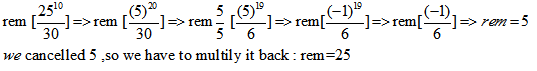This is the final article covering some more handy methods to find remainders, out of which the most comfortable method can be chosen to save time dealing with remainder questions.

Method 1:

Example: Suppose we have two numbers “a” and “b”. If “a” is in form of 7n +4 and “b” is in form of 7m+1.

Find remainder when a+b is divided by 7.

Given a = 7n +4

and b = 7m + 1

So when we add them we will have a +b = 7n + 7m + 4 + 1 = 7 (n + m) + 5

That means we have a multiple of 7 and a remainder 5, because five is smaller than the 7. So it cannot be divided further and hence this 5 is our remainder.

Difference

What will be the remainder when a – b is divided by 7?

Given a = 7n +4

and b = 7m + 1

So when we subtract them we will have a – b= (7n +4) – (7m + 1)

= 7(n – m ) + 4 – 1

=  7(n – m ) + 3.

That means we will have a multiple of 7 with addition of 3 and because three

is smaller than the 7 so it cannot be divided further and hence this 3 is our remainder .

Multiplication

What will be the remainder when a x b is divided by 7?

Given: a = 7n +4

and b = 7m + 1

So when we multiply them we will have a x b  = (7n +4) x (7m + 1)

= 7n(7m + 1)  + 4(7m + 1)

= 49nm + 7n  + 28nm + 4. Here we can see that each and every number is a multiple of 7 except 4. Hence this 4 is our remainder.

Some Important Properties of Prime Numbers

• Any single digit number written (P – 1) times is divisible by P, where P is a prime number > 5.

Example: Find the remainder of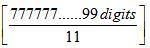Solution:

11 is a prime number. Any digit repeated (11-1) times or multiple of 10   times must be divisible by 11.

Now, in the given question, digit 7 is repeated 99  times hence it must be divisible by 11 .so remainder = 0

Example: Find the remainder of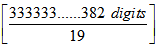Solution:

19 is a prime number and any digit repeated (19-1) times or multiple of 18   times must be divisible by 19.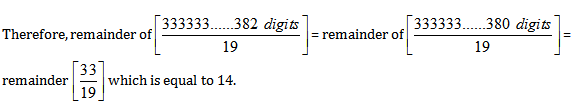Method 2: Cancelation

Cancellation is applied to make the calculations easier. First we cancel out the common factors and then multiply those factors back after finding remainders.

Example: Find the remainder of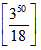Solution:

Method 1:

Since common factor is 9 = 32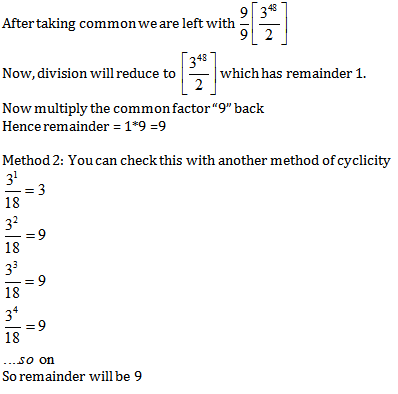Try out some more questions based on this concept so as to get a good hold of this topic.

EXERCISE:

Question 1. What is the remainder when 111 + 68 + 48 is divided by 11?
a) 5
b) 7
c) 9
d) None

### Answer and Explanation

Solution: option b 111 leaves remainder 1 when divided by 11

68 leaves remainder 2 when divided by 11

48 leaves remainder 4 when divided by 11

Thus111 + 68 + 48 leaves remainder 1+2+4 =7 when divided by 11

Question 2. Find the remainder of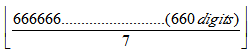a)5
b)0
c)9
d)None

### Answer and Explanation

7  is a prime number.  Any digit repeated (7-1)=6 times or multiple of 6 times must be divisible by 7.

Now, in the given question digit 6 is repeated 660 times hence it must be divisible by 7, so remainder = 0

Question 3.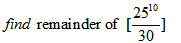a) 25
b) 0
c) 50
d) None

### Answer and Explanation

Solution: option a